# May 27th Electronics TV's & Laptops

### Auction Details

Electronics TV's & Laptops
Choose Your Layout >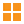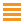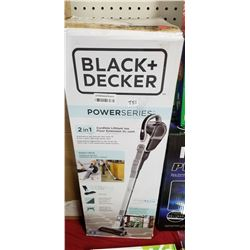51 - Shelf One
CDC Auctions
Shelf One
Bidding Has Concluded

Sold to f***r for (55.00 + 7.15) x 1 = 62.15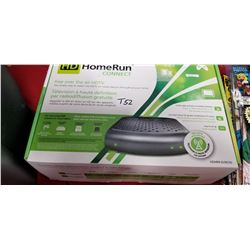52 - Shelf Two
CDC Auctions
Shelf Two
Bidding Has Concluded

Sold to f***r for (15.00 + 1.95) x 1 = 16.95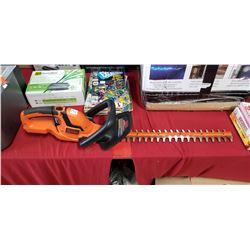53 - Shelf One
CDC Auctions
Shelf One
Bidding Has Concluded

Sold to f***r for (15.00 + 1.95) x 1 = 16.95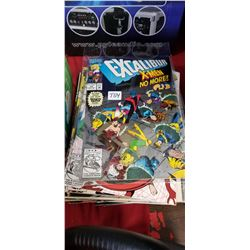54 - Shelf One
CDC Auctions
Shelf One
Bidding Has Concluded

Sold to d*******M for (22.50 + 2.93) x 1 = 25.43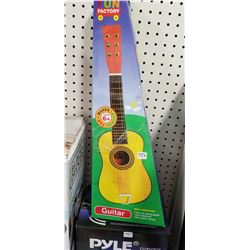56 - Shelf One
CDC Auctions
Shelf One
Bidding Has Concluded

Sold to f***r for (7.50 + 0.98) x 1 = 8.48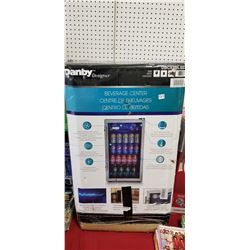63 - Danby Beverage Center
CDC Auctions
Shelf One
Bidding Has Concluded

Sold to c********a.. for (95.00 + 12.35) x 1 = 107.35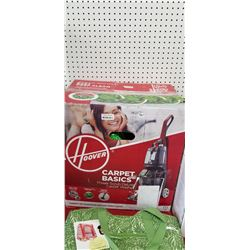65 - Shelf One
CDC Auctions
Shelf One
Bidding Has Concluded

Sold to f***r for (22.50 + 2.93) x 1 = 25.43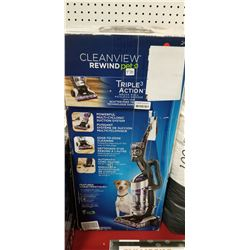70 - Shelf Two
CDC Auctions
Shelf Two
Bidding Has Concluded

Sold to f***r for (22.50 + 2.93) x 1 = 25.43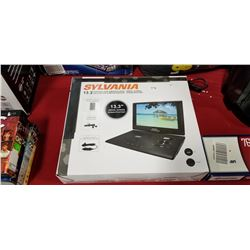71 - Shelf One
CDC Auctions
Shelf One
Bidding Has Concluded

Sold to f***r for (22.50 + 2.93) x 1 = 25.43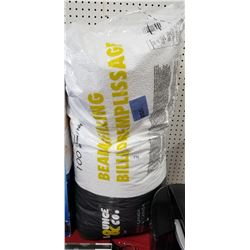72 - Shelf One
CDC Auctions
Shelf One
Bidding Has Concluded

Sold to f***r for (5.00 + 0.65) x 1 = 5.6573 - Shelf One
CDC Auctions
Shelf One
Bidding Has Concluded

Sold to f***r for (12.50 + 1.63) x 1 = 14.13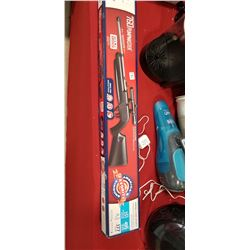80 - Shelf One
CDC Auctions
Shelf One
Bidding Has Concluded

Sold to N******a for (50.00 + 6.50) x 1 = 56.50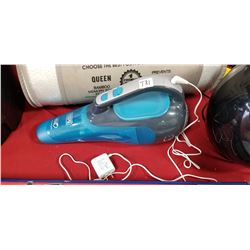81 - Shelf One
CDC Auctions
Shelf One
Bidding Has Concluded

Sold to f***r for (20.00 + 2.60) x 1 = 22.60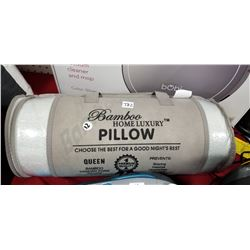82 - Shelf One
CDC Auctions
Shelf One
Bidding Has Concluded

Sold to f***r for (12.50 + 1.63) x 1 = 14.13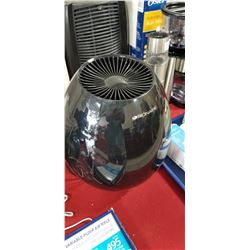83 - Shelf One
CDC Auctions
Shelf One
Bidding Has Concluded

Sold to f***r for (7.50 + 0.98) x 1 = 8.48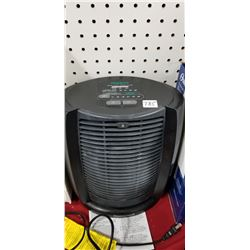85 - Shelf One
CDC Auctions
Shelf One
Bidding Has Concluded

Sold to f***r for (22.50 + 2.93) x 1 = 25.43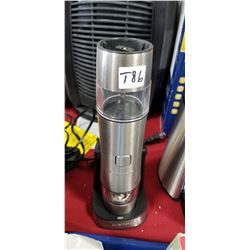86 - Shelf One
CDC Auctions
Shelf One
Bidding Has Concluded

Sold to f***r for (5.00 + 0.65) x 1 = 5.65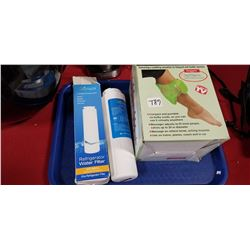87 - Shelf One
CDC Auctions
Shelf One
Bidding Has Concluded

Sold to f***r for (5.00 + 0.65) x 1 = 5.65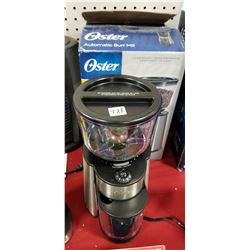88 - Shelf Two
CDC Auctions
Shelf Two
Bidding Has Concluded

Sold to f***r for (12.50 + 1.63) x 1 = 14.13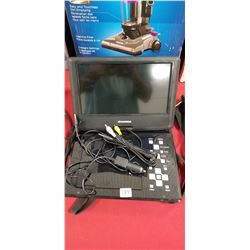89 - Shelf One
CDC Auctions
Shelf One
Bidding Has Concluded

Sold to f***r for (17.50 + 2.28) x 1 = 19.78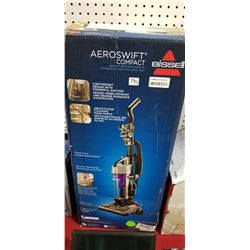90 - Shelf One
CDC Auctions
Shelf One
Bidding Has Concluded

Sold to f***r for (22.50 + 2.93) x 1 = 25.43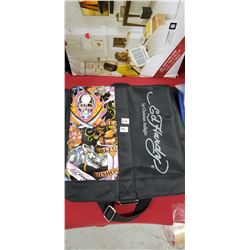91 - Shelf One
CDC Auctions
Shelf One
Bidding Has Concluded

Sold to f***r for (5.00 + 0.65) x 1 = 5.65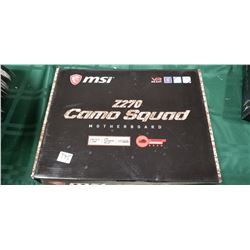95 - Shelf One
CDC Auctions
Shelf One
Bidding Has Concluded

Sold to f***r for (32.50 + 4.23) x 1 = 36.73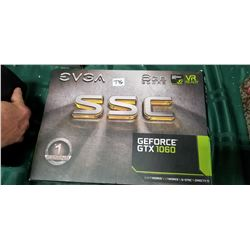96 - Shelf One
CDC Auctions
Shelf One
Bidding Has Concluded

Sold to f***r for (200.00 + 26.00) x 1 = 226.00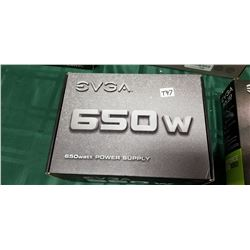97 - Shelf One
CDC Auctions
Shelf One
Bidding Has Concluded

Sold to f***r for (17.50 + 2.28) x 1 = 19.78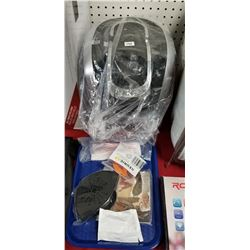98 - Shelf One
CDC Auctions
Shelf One
Bidding Has Concluded

Sold to f***r for (10.00 + 1.30) x 1 = 11.30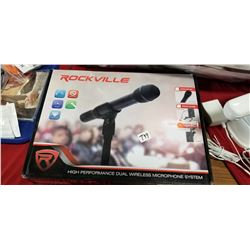99 - Shelf One
CDC Auctions
Shelf One
Bidding Has Concluded

Sold to f***r for (40.00 + 5.20) x 1 = 45.20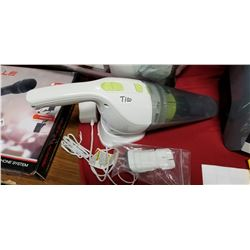100 - Shelf One
CDC Auctions
Shelf One
Bidding Has Concluded

Sold to f***r for (7.50 + 0.98) x 1 = 8.48